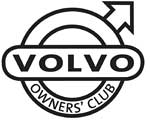# Volvo XC90 VIN Plates

1800  |  200  |  300  |  400  |  700  |  850 - 900  |  40/50  |  60  |  70  |  80  |  90  |  XC90

This information will help you to understand the information on the VIN plate of your Volvo car.

Click on the model series number above

Example VIN Number:

YV1CN59G432123456

YV1 C SN 59 G 4 3 2 123456
Manufacturer Code Vehicle Type Type Engine Type Emission Gearbox Model Year Factory Code Chassis Number

Manufacturer Code
YV1 = cars YV2 = trucks YV3 = buses YV4 = multipurpose 4V1 = trucks 4V2 = 4V3 =
4V4 = trucks 4V5 = trucks 4V6 = 4VL = 4VM = 4VZ = MHA = PT. Central Sole Agency

Vehicle Type
C = XC90

Type
M = XC90 AWD 5 sea N = XC90 2WD 5 seat Y = XC90 2WD 7 seat Z = XC90 AWD 7 seat

Engine Type
59 = B5254T2 79 = D5244T 91 = B6294T

Emissions
3 = KOD: EM F1 9 = KOD: EM F2 B = KOD: EM X3 G = KOD: EM X1 EXC (USA)(CDN) H = KOD: EM X2 (USA)(CDN) J = KOD: EM X5 EXC (USA)(CDN) Z = KOD: EM X6 (USA)(CDN) W = KOD: EM Z4

Gearbox
4 = M66AWD manual gearbox 7 = AW50AWD automatic gearbox 8 = 4T65AWD automatic gearbox

Model Year From 1980
A = 1980 B = 1981 C = 1982 D = 1983 E = 1984 F = 1985 G = 1986 H = 1987 J = 1988 K = 1989 L = 1990 M = 1991
N = 1992 P = 1993 R = 1994 S = 1995 T = 1996 V = 1997 W = 1998 X = 1999 Y = 2000 1 = 2001 2 = 2002 3 = 2003
4 = 2004 5 = 2005 6 = 2006 7 = 2007 8 = 2008 9 = 2009 A = 2010 (code restarted) B = 2011 C = 2012 D = 2013 E = 2014 ---

Factory Code
0 = Sweden, Kalmar Plant 1 = Sweden, Torslanda Plant VCT 21(Volvo Torslandaverken) (Gothenburg) 2 = Belgium, Ghent Plant VCG 22 3 = Canada, Hatdfax Plant 4 = Italy, - Bertone models 240 5 = Malaysia, 6 = Australia,
7 = Indonesia, A = Sweden, Uddevalla Plant (Volvo Cars/TWR (Tom Walkinshaw Racing)) B = Italy, - Bertone Chongq 31 D = Italy, - Bertone models 780 E = Singapore, F = The Netherlands, Born Plant (NEDCAR) J = Sweden, Uddevalla Plant VCU 38 (Volvo Cars/ Pininfarina Sverige AB)